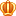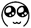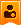##账号 自动登录 找回密码 密码 立即注册
 搜索

# [已解决]请教大神发表于 5 天前 | 显示全部楼层 |阅读模式

### 马上注册，结交更多好友，享用更多功能^_^

x5 天前

1. import random

2. def has_same_birthday():
3.     """判断23个人中是否至少有两个人生日相同"""
4.     birthdays = [random.randint(1, 365) for _ in range(23)]
5.     return len(set(birthdays)) < 23

6. def estimate_probability(num_samples):
7.     """根据给定的样本数量估计概率"""
8.     num_success = sum(has_same_birthday() for _ in range(num_samples))
9.     return num_success / num_samples

10. # 计算在不同样本数量下的概率
11. for num_samples in [1000, 10000, 100000]:
12.     probability = estimate_probability(num_samples)
13.     print(f"样本数量为{num_samples}时，23个人中至少两个人生日相同的概率为{probability:.2%}")发表于 5 天前 | 显示全部楼层    本楼为最佳答案
 .pct{min-height:100px;}我们可以通过模拟的方式来计算出在不同的样本数量下，23个人中至少两个人生日相同的概率。具体方法是随机生成很多个23个人的生日组合进行计算，并统计其中至少存在两个人生日相同的组合数占总组合数的比例。下面是一个示例代码： import random def has_same_birthday():     """判断23个人中是否至少有两个人生日相同"""     birthdays = [random.randint(1, 365) for _ in range(23)]     return len(set(birthdays)) < 23 def estimate_probability(num_samples):     """根据给定的样本数量估计概率"""     num_success = sum(has_same_birthday() for _ in range(num_samples))     return num_success / num_samples # 计算在不同样本数量下的概率 for num_samples in [1000, 10000, 100000]:     probability = estimate_probability(num_samples)     print(f"样本数量为{num_samples}时，23个人中至少两个人生日相同的概率为{probability:.2%}")复制代码 上述代码中， has_same_birthday() 函数用于判断 23 个人中是否至少有两个人生日相同，具体做法是随机生成23个整数（对应生日），并使用 set() 函数去重，如果集合的长度小于23，则说明至少有两个人生日相同。estimate_probability() 函数则用于根据给定的样本数量估计概率，具体做法是循环生成指定数量的样本，并计算其中至少存在两个人生日相同的概率。最后，在样本数量分别为 1000、10000、100000 时，我们输出了概率的估计结果。 有用请设置最佳答案楼主| 发表于 5 天前 | 显示全部楼层
 谢谢哦

 您需要登录后才可以回帖 登录 | 立即注册 本版积分规则 回帖后跳转到最后一页

GMT+8, 2023-5-31 09:09